Author: Oscar Cronquist Article last updated on February 03, 2023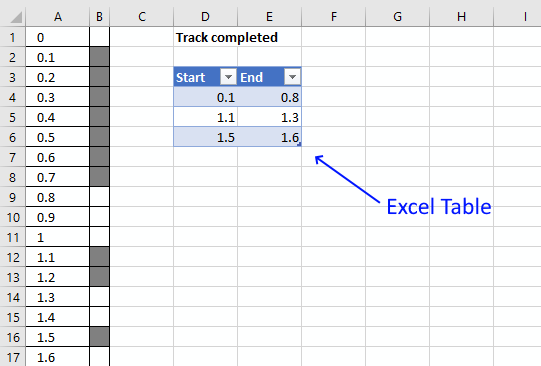This article demonstrates a Conditional Formatting formula that lets you highlight cells based on numerical ranges specified in an Excel Table.

Column A contains numbers from 0 (zero) to 100 in steps of 0.1. Cells in column B are highlighted if the number is inside a range specified in the Excel Table.

For example, cell A1 contains 0 (zero), the corresponding cell B1 is not highlighted. Value 0 (zero) is not in any of the three ranges specified in cell range D4:E6. They are 0.1-0.8, 1.1-1.3, and 1.4-1.6.

I am working on a railway project as planner. How can I create a dynamic strip chart in Excel?
Assume the total length is 10 kilometers and each cell of 100 meters.
If I update the progress from 9.1 km to 9.8 km in the table, how the cell gets highlighted corresponding to the entered chainages.

### Conditional Formatting formula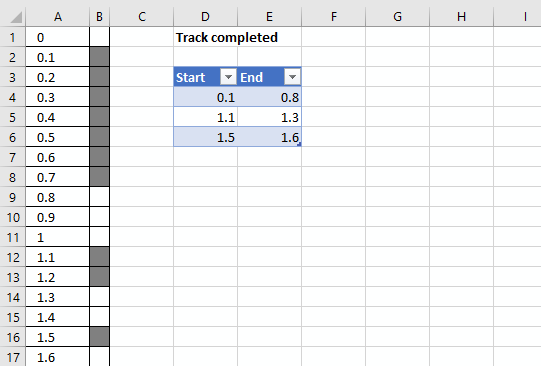The worksheet above shows you km in column A and corresponding highlighted cells in column B if they are specified in the Excel Table.

You can easily add or remove values to the Excel Table without the need to adjust the conditional formatting formulas.

Conditional formatting formula applied to cell range B1:B101:

=SUMPRODUCT((A1<INDIRECT("Table1[End]"))*(A1>=INDIRECT("Table1[Start]")))

### How to create an Excel Table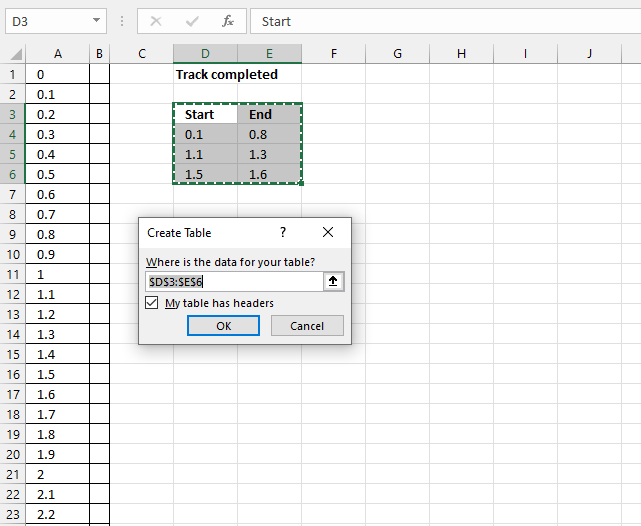1. Select cell range D3:E6
2. Go to tab "Insert" on the ribbon
3. Press with left mouse button on the "Table" button

Tip! You can also use the shortcut key CTRL + T to create a table

Excel Tables let you easily organize, filter, and format data on a worksheet. They also let you use structured references that can gro without the need to adjust cell references in a formula.

### How to apply a conditional formatting formula to a cell range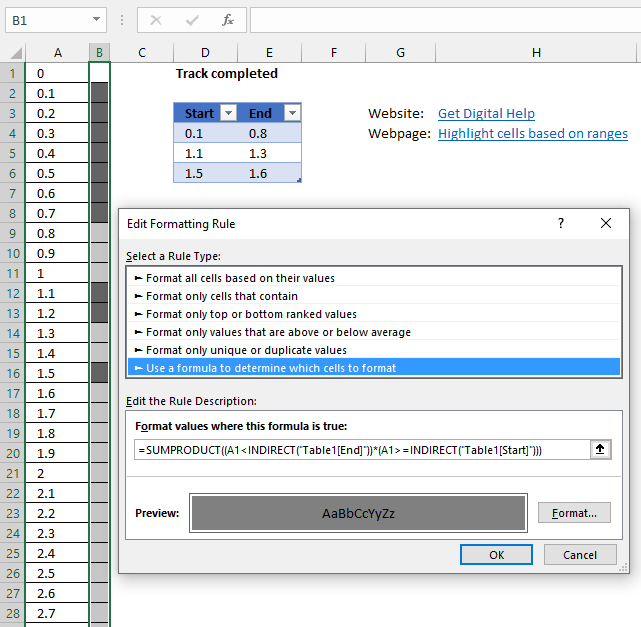1. Select cell range B1:B101.
2. Go to tab "Home" on the ribbon if you are not already there.
3. Press with mouse on the "Conditional Formatting" button to expand a menu.
4. Press with left mouse button on "New Rule..".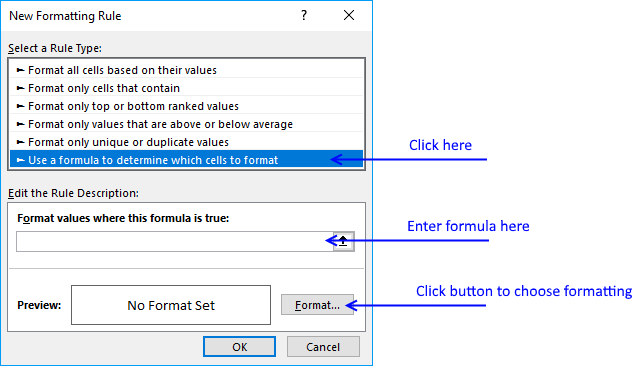5. Press with left mouse button on "Use a formula to determine which cells to format".
6. Type above formula in dialog box formula bar.
7. Press with left mouse button on the "Format..." button.
8. Go to tab "Fill".
9. Pick a fill color.
10. Press with left mouse button on the OK button.
11. Press with left mouse button on the OK button.

### Explaining conditional formatting formula

You can't use structured references in a conditional formatting formula unless you use the INDIRECT function for each reference. This applies to Data Validation Lists as well.

#### Step 1 - Reference an Excel Table in a Conditional Formatting formula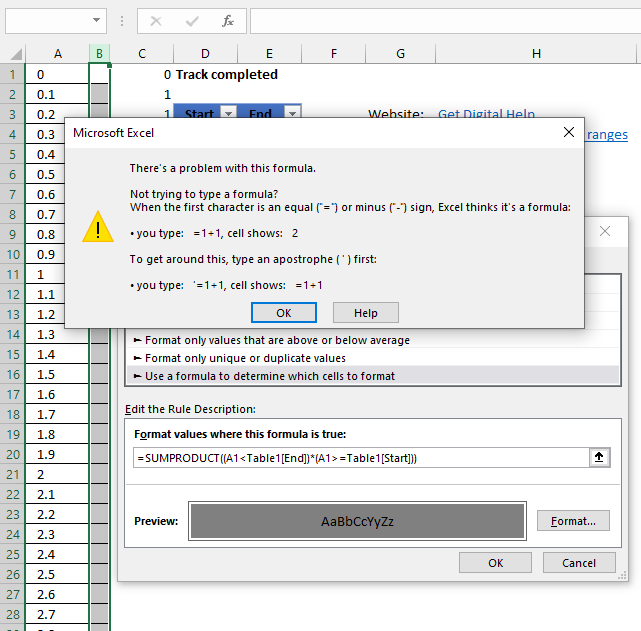Excel returns an error message if you try to use a reference to an Excel Table in a Conditional Formatting formula.

There is a workaround, simply use the INDIRECT function to make this possible.

Table1[End]

becomes

INDIRECT("Table1[End]")

Remember to use the INDIRECT function for all instances where you reference an Excel Table.

#### Step 2 - Check if current cell value is less than the END range values in the Excel Table

(A1<INDIRECT("Table1[End]")

becomes

0<{0.8;1.3;1.6}

and returns {TRUE;TRUE;TRUE}

#### Step 3 - Check if current cell value is greater than or equal to the START values in the Excel Table

A1>=INDIRECT("Table1[Start]"

becomes

0>={0.1;1.1;1.5}

and returns {FALSE;FALSE;FALSE}

#### Step 4 - Multiply arrays

(A1<INDIRECT("Table1[End]"))*(A1>=INDIRECT("Table1[Start]"))

becomes

{TRUE;TRUE;TRUE}*{FALSE;FALSE;FALSE}

and returns {0;0;0}

Multiplying boolean values creates integers.

#### Step 5 - Sum array

SUMPRODUCT((A1<INDIRECT("Table1[End]"))*(A1>=INDIRECT("Table1[Start]")))

becomes

SUMPRODUCT( {0;0;0})

and returns 0.

0 means False so cell B1 is not formatted gray.